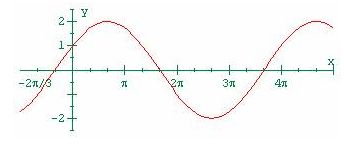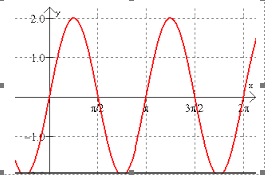#### MAT-HS.F-IF.07.f

 MAT-HS Targeted Standards(F) Concept: Function(IF) Domain: Interpreting FunctionsCluster: Analyze functions using different representations MAT-HS.F-IF.07* Graph functions expressed symbolically and show key features of the graph, by hand in simple cases and using technology for more complicated cases. a. Graph linear and quadratic functions and show intercepts, maxima, and minima. b. Graph square root, cube root, and piecewise-defined functions, including step functions and absolute value functions. c. Graph polynomial functions, identifying zeros when suitable factorizations are available, and showing end behavior. d. (+) Graph rational functions, identifying zeros and asymptotes when suitable factorizations are available, and showing end behavior. e. Graph exponential and logarithmic functions, showing intercepts and end behavior, and trigonometric functions, showing period, midline, and amplitude. f.  Graph f(x)=sin x and f(x)=cos x as representations of periodic phenomena. g.  (+) Graph trigonometric functions, showing period, midline, phase shift and amplitude

• I can
• I can

• I can
• I can

• I can
• I can
• I can

• I can
• I can

## Alg II Graph Sine and Cosine Functions Proficiency Scale

Score   Description Sample Activity

4.0

In addition to Score 3.0, the student demonstrates in-depth inferences and applications regarding more complex material that go beyond end of instruction expectations. Write an equation for the graph in the form y = A sin ( Bx + C ). Write an equation for the same graph in the form y = A cos( Bx+C).3.5 In addition to Score 3.0 performance, the student demonstrates in-depth inferences and applications regarding the more complex content with partial success.

3.0

(proficient)

The student can:
• graph trigonometric functions expressed symbolically and show key features of the graph (graph simple cases by hand and use technology to show more complicated cases)

The student exhibits no major errors or omissions.
Graph the following equations and identify all the key features including: increasing interval, decreasing interval, maximums, minimums, domain and range, amplitude, increment, period, midline. f(x) = asinx

2.5 The student demonstrates no major errors or omissions regarding the simpler details and processes (Score 2.0 content) and partial knowledge of the more complex ideas and processes (Score 3.0 content).

2.0

(progressing)

There are no major errors or omissions regarding the simpler details and processes as the student can:

• recognize and recall basic terminology, such as:

• trigonometric function

• amplitude

• increment

• period

• midline

• domain

• range

However, the student exhibits major errors or omissions regarding the more complex ideas and processes.

 Given the graph, identify all the key features including: increasing interval, decreasing interval, maximums, minimums, domain and range, amplitude, increment, period,and midline.1.5 The student demonstrates partial knowledge of the simpler details and processes (Score 2.0 content) but exhibits major errors or omissions regarding the more complex ideas and procedures (Score 3.0 content).

1.0

(beginning)

With help, the student demonstrates a partial understanding of some of the simpler details and processes (Score 2.0 content) and some of the more complex ideas and processes (Score 3.0 content).
0.5 With help, the student demonstrates a partial understanding of some of the simpler details and processes (Score 2.0 content) but not the more complex ideas and processes (Score 3.0 content).
0.0 Even with help, the student demonstrates no understanding or skill.

## Resources

 Web Vocab